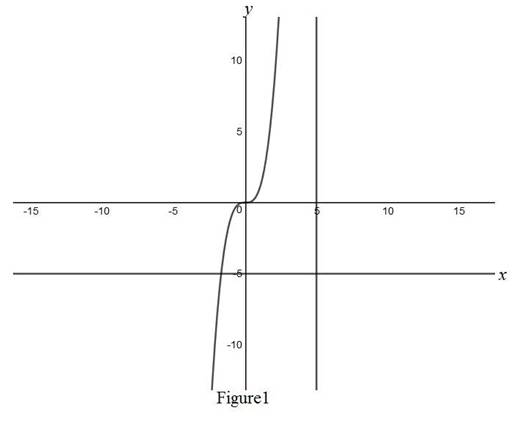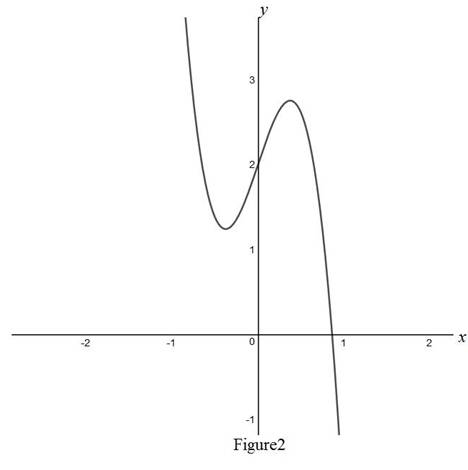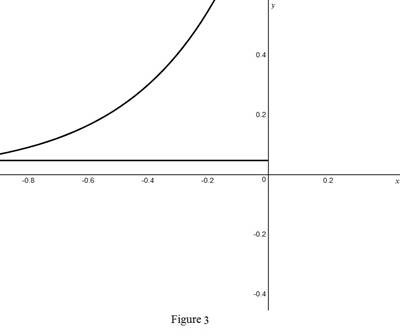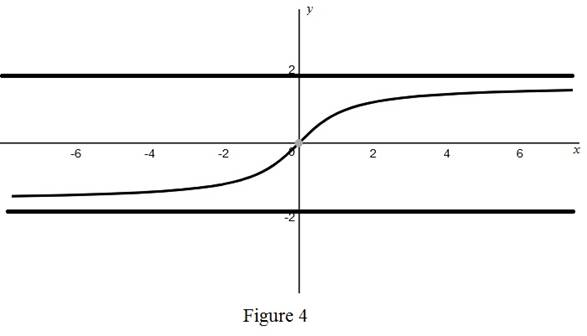# The graph of y = f ( x ) intersect vertical and horizontal asymptote.### Single Variable Calculus: Concepts...

4th Edition
James Stewart
Publisher: Cengage Learning
ISBN: 9781337687805### Single Variable Calculus: Concepts...

4th Edition
James Stewart
Publisher: Cengage Learning
ISBN: 9781337687805

#### Solutions

Chapter 2.5, Problem 2E

(a)

To determine

## To find: The graph of y=f(x) intersect vertical and horizontal asymptote.

Expert Solution

The graph of y=f(x) cannot intersect vertical asymptote and it may insect horizontal asymptote.

### Explanation of Solution

Graph:From Figure 1, it is clear that the graph of y=f(x) cannot intersect vertical asymptote and it may intersect horizontal asymptote.

(b)

To determine

### To find: The possibility number of horizontal asymptotes in the graph.

Expert Solution

There are one or two horizontal asymptotes in the graph or no horizontal asymptote also possible.

### Explanation of Solution

Graph:Generally, no horizontal asymptote for polynomial degree one or higher degree.

Graph:Generally, one horizontal for exponential functions.

Graph:Generally, two horizontal asymptotes for inverse tangent functions.

Therefore, horizontal asymptotes exists for limxf(x) and limxf(x).

For the function y=f(x) cannot have more than two horizontal asymptotes.

Thus, from graphs it is observed that there is one or two horizontal asymptote in the graph or no horizontal asymptote also possible.

### Have a homework question?

Subscribe to bartleby learn! Ask subject matter experts 30 homework questions each month. Plus, you’ll have access to millions of step-by-step textbook answers!